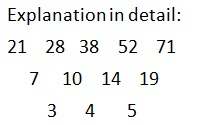# Quantitative Aptitude Quiz 4Quantitative Aptitude Quiz 3 consists of 10 new pattern questions asked in SBI PO, SBI Clerk, IBPS PO, IBPS Clerk exams. Candidates can practice such new pattern questions to get familiar with the actual type of questions asked in IBPS, SBI, and other insurance exams:

Question 1: What should come in place of question mark (?) in the following number series?
19, 30, 48, 96, ?, 439

a. 113
b. 244
c. 143
d. 210
e. None of the above

d. 210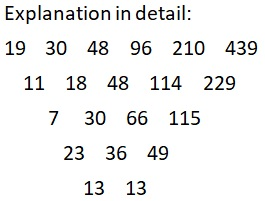Question 2: In each of the following questions, two equations I and II are given. Solve both the equations and given answer: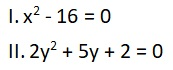a. x is greater than y
b. x is less than equal to y
c. x is less than y
d. x is greater than equal to y
e. x = y or relationship between x and y can’t be determined

e. x = y or relationship between x and y can’t be determined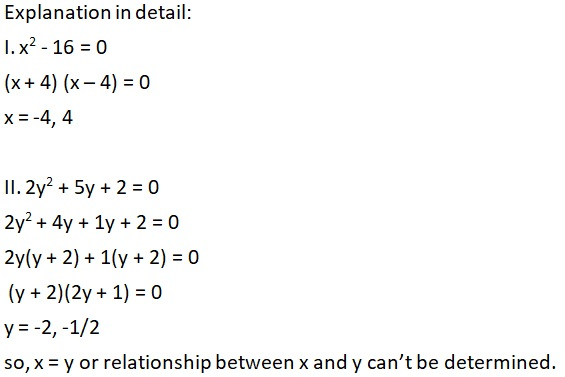Question 3: In each of the following questions, two equations I and II are given. Solve both the equations and given answer: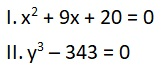a. x is greater than y
b. x is less than equal to y
c. x is less than y
d. x is greater than equal to y
e. x = y or relationship between x and y can’t be determined

c. x is less than y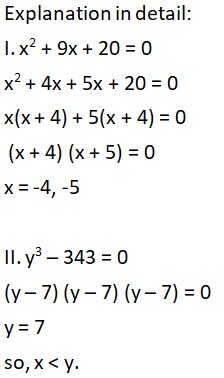Question 4: In each of the following questions, two equations I and II are given. Solve both the equations and given answer: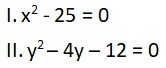a. x is greater than y
b. x is less than equal to y
c. x is less than y
d. x is greater than equal to y
e. x = y or relationship between x and y can’t be determined

e. x = y or relationship between x and y can’t be determined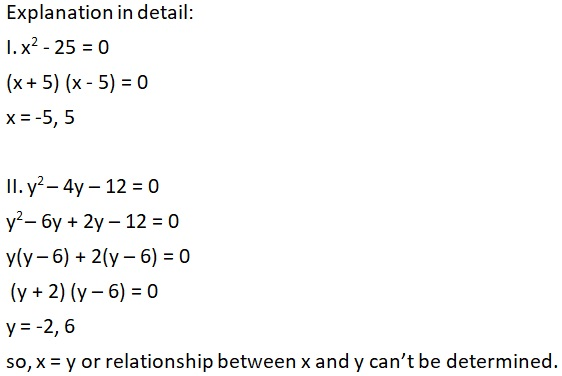Question 5: In each of the following questions, two equations I and II are given. Solve both the equations and given answer: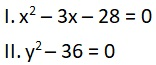a. x is greater than y
b. x is less than equal to y
c. x is less than y
d. x is greater than equal to y
e. x = y or relationship between x and y can’t be determined

e. x = y or relationship between x and y can’t be determined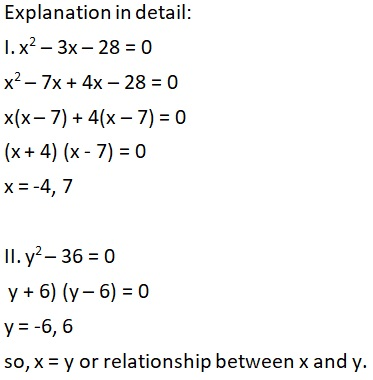Question 6: In each of the following questions, two equations I and II are given. Solve both the equations and given answer: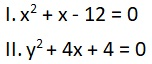a. x is greater than y
b. x is less than equal to y
c. x is less than y
d. x is greater than equal to y
e. x = y or relationship between x and y can’t be determined

e. x = y or relationship between x and y can’t be determined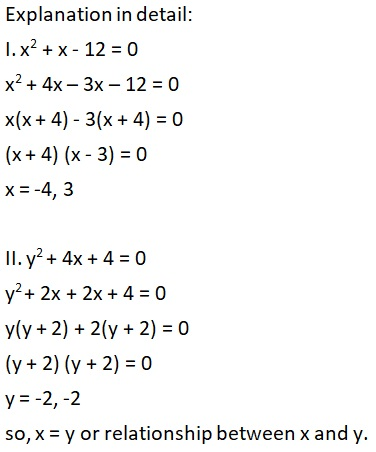Question 7: When 6% of 350 is subtracted from 8% of 450, the value obtained is ___ less than 33.

a. 18
b. 28
c. 15
d. 22
e. None of the above

a. 18
Explanation in detail:
The expression can be written as:
8% of 450 – 6% of 350 = 33 – ?
? = 33 – (8% of 450 – 6% of 350)
? = 33 – (36 – 21)
?= 33 – 15 = 18

Question 8: What value should come in the place of the question mark (?) in the given question?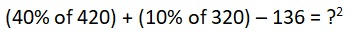a. 4
b. 8
c. 7
d. 6
e. 9

b. 8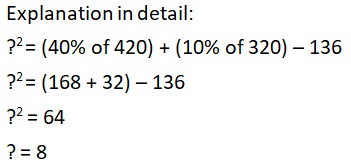Question 9: What should come in place of question mark (?) in the following number series?
37, 46, 30, 55, ?

a. 21
b. 23
c. 17
d. 19
e. None of the above

d. 19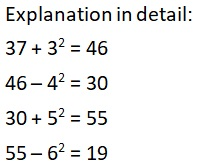Question 10: What should come in place of question mark (?) in the following number series?
21, 28, 38, 52, ?

a. 70
b. 61
c. 81
d. 71
e. None of the above

d. 71